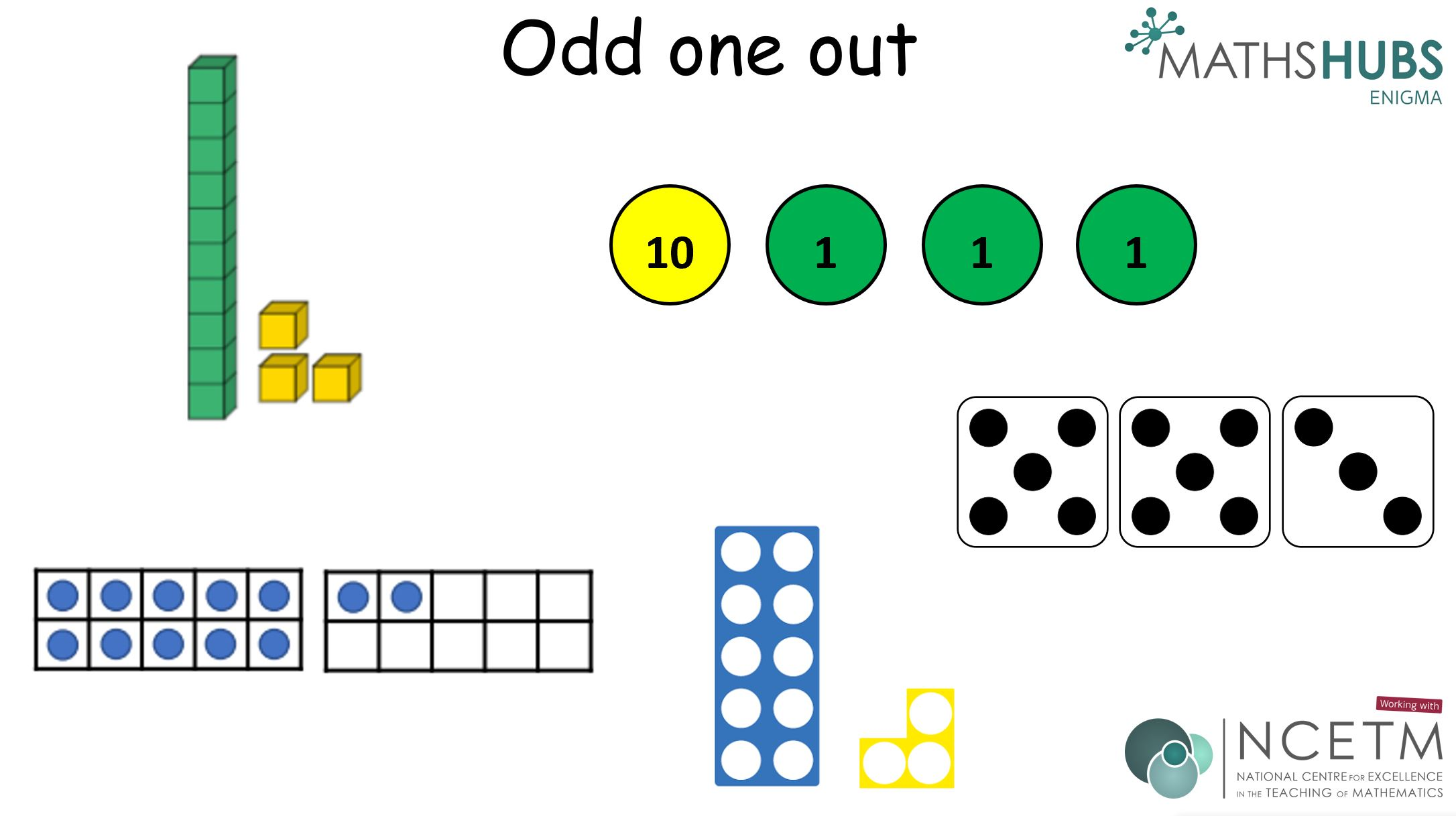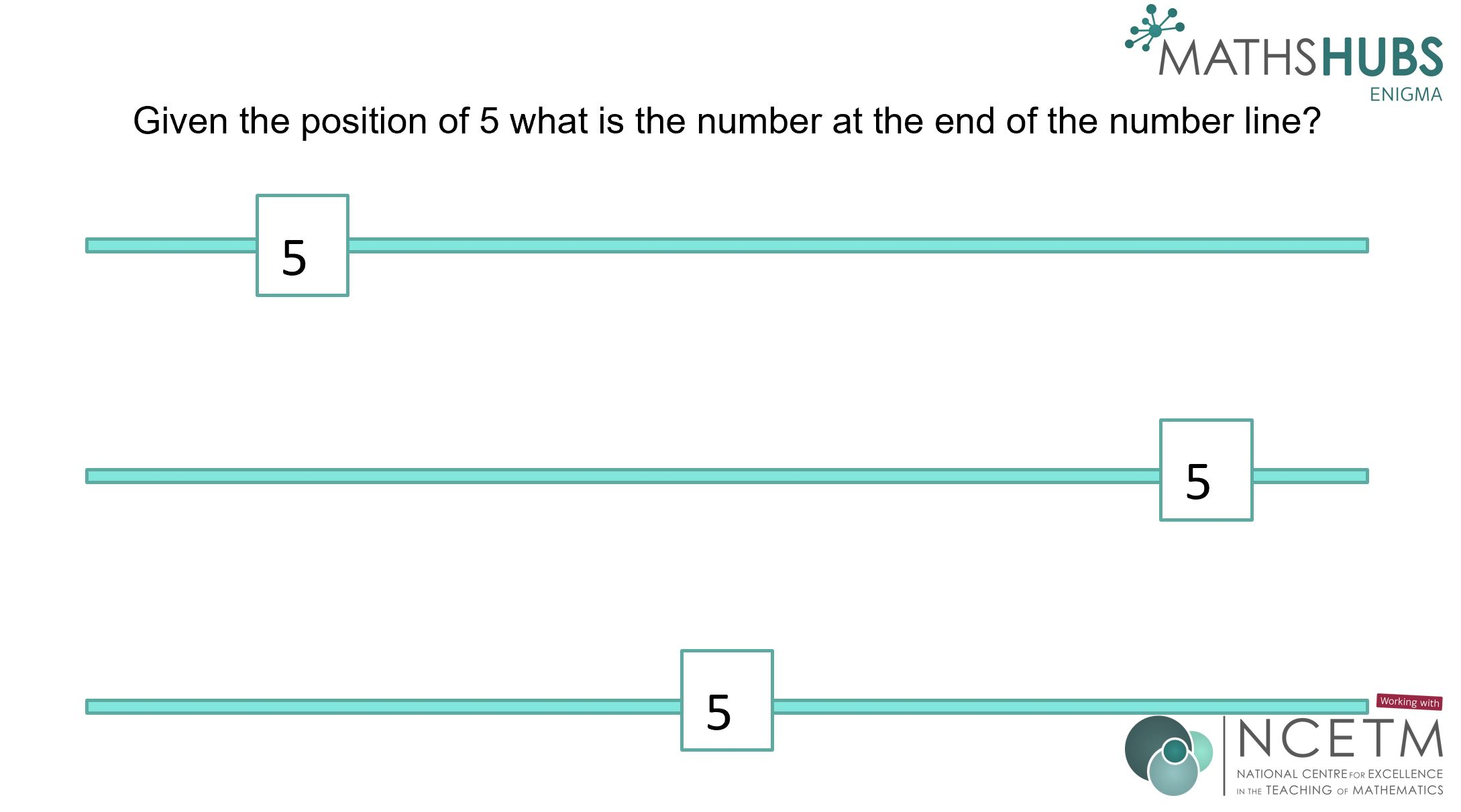# Mathematical Thinking

Encouraging and enabling our pupils to think mathematically and to be able to apply their mathematical learning. In order to enable our pupils to be equipped for their future learning and lives our pupils need to be able to think deeply. Mathematical thinking links with the aims of the National Curriculum – Reasoning and Problem-Solving.
••# Bank of Support

The NRICH website has a wealth of resources to aid and further develop mathematical thinking and learning. Definitely worth exploring.
Is there a shared understanding of mathematical reasoning at your school? Greg McGill at Swallowfield school shares a staff meeting from his school.
List of reasoning strategies to develop mathematical thinking
Adaptable slides to develop mathematical thinking relating to multiplication and division.
Mathematical thinking in Geometry – Some examples to try
Mathematical thinking with addition and subtraction – some ideas to try